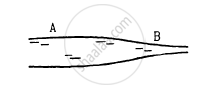Advertisement Remove all ads

# Water Flows Through a Horizontal Tube of Variable Cross Section. the Area of Cross Section at a and B Are 4 Mm2 and 2 Mm2 Respectively. If 1 Cc of Water Enters per Second Through A, - Physics

Sum

Water flows through a horizontal tube of variable cross section. The area of cross section at A and B are 4 mm2 and 2 mm2 respectively. If 1 cc of water enters per second through A, find (a) the speed of water at A, (b) the speed of water at B and (c) the pressure difference PA − PB.Advertisement Remove all ads

#### Solution

(a) Given:
The areas of cross-sections of the tube at A and B are 4 mm2 and 2 mm2, respectively.
1 cc of water enters per second through A.
Let:

$\rho$= Density of the liquid
PA = Pressure of liquid at A
PB = Pressure of liquid at B
(a)$\frac{Q_A}{t} = \frac{1a}{s} = \text{ Discharge }$
$\Rightarrow a_A \times V_A = Q_A$
$\text{ Given: }$
$a_A = 4 {\text{ mm }}^2 = 4 \times {10}^{- 2} {\text{cm}}^2$
$\therefore 4 \times {10}^{- 2} \times V_A = 1\text{cc/s}$
$\Rightarrow V_A = 25 \text{ m/s}$
$(b) a_A \times V_A = a_B \times V_B$
$\Rightarrow 4 \times {10}^{- 2} \times 25 = 2 \times {10}^{- 2} \times V_B$
$\Rightarrow V_B = 50 \text{ cm/s}$
(c) By Bernoulli's equation, we have:

$\frac{1}{2}\rho \text{v}_A^2 + P_A = \frac{1}{2}\rho \text{ v}_B^2 + P_B$
$\Rightarrow ( P_A - P_B ) = \frac{1}{2}\rho\left( v_B^2 - \text{v}_A^2 \right)$
$= \frac{1}{2} \times 1 \times (2500 - 625)$
$= \frac{1875}{2} = 937 . 5 \text{dyn/ {cm}}^2$
$= 93 . 75 \text{N/ m}^2$
Is there an error in this question or solution?
Advertisement Remove all ads

#### APPEARS IN

HC Verma Class 11, 12 Concepts of Physics 1
Chapter 13 Fluid Mechanics
Q 28 | Page 275
Advertisement Remove all ads

#### Video TutorialsVIEW ALL 

Advertisement Remove all ads
Share
Notifications

View all notifications

Forgot password?